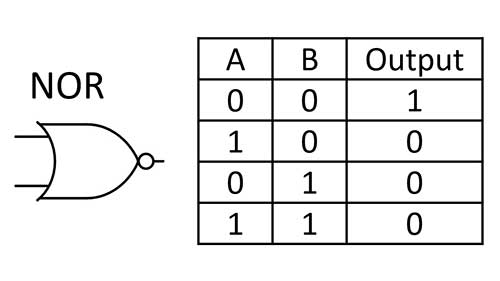# NOR Gate

The NOR gate is a universal logic gate that can be built using only two transistors. It can be thought of as the not OR gate or having inverted logic to the OR gate. This is a useful logic gate as it was the core logic gate used in the Apollo Guidance Computer. There were two versions of the computer, the first used 4,100 NOR gates with each gate being built on separate integrated circuits. This was the first computer based on silicon-based integrated circuits. The second version used 2,800 integrated circuits which mostly had two NOR gates on each IC.The circuit above shows a NOR gate that is built on a breadboard using two transistors, three resistors, three ground wires, one jumper wire, and a yellow LED. Power is supplied with a 5-volt external battery pack. Both inputs A and B are on which is why the output is off. The only case where the output is on is when both inputs A and B are off.

This video shows how to build all types of logic gates. At 38:08 the NOR gate is discussed in detail. How to build an AND gate, OR gate, and XOR gate with NOR gates is also demonstrated.  A NAND gate can also be built with NOR gates but that would not be used very often as a NAND gate can also be built with two transistors.

## NOR Gate Truth TableThe NOR gate symbol and truth table are shown above. Its symbol looks like an OR gate but has a circle to illustrate that it has inverted logic. The truth table makes it clear that the output is on when both inputs A and B are off. In all other cases, one or more inputs will be on and the output will be off. This is the opposite logic of an OR gate.

## NOR Gate Circuit DiagramThe circuit above shows a simple way to build a NOR gate. How each connection between the collector, base, and emitter of each transistor is made with the resistors and ground is clearly shown. This is not the only way to make a NOR gate though as four NAND gates can also be used to build a NOR gate. Since the NOR gate is a universal digital logic gate it can be used to make all the other types of logic gates.